## Where are we so far?

1. Introduction: vignette("gcplyr")
2. Importing and transforming data: vignette("import_transform")
3. Incorporating design information: vignette("incorporate_designs")
4. Pre-processing and plotting your data: vignette("preprocess_plot")
5. Processing your data: vignette("process")
6. Analyzing your data: vignette("analyze")
7. Dealing with noise: vignette("noise")
8. Statistics, merging other data, and other resources: vignette("conclusion")

So far, we’ve imported and transformed our measures, combined them with our design information, pre-processed, processed, and plotted our data. Now we’re going to analyze our data by summarizing our growth curves into a number of metrics.

library(gcplyr)
#> ##
#> ## gcplyr (Version 1.6.0, Build Date: 2023-09-13)
#> ## See http://github.com/mikeblazanin/gcplyr for additional documentation
#> ## Please cite software as:
#> ##   Blazanin, Michael. 2023. gcplyr: an R package for microbial growth
#> ##   curve data analysis. bioRxiv doi: 10.1101/2023.04.30.538883
#> ##

library(dplyr)
#>
#> Attaching package: 'dplyr'
#> The following objects are masked from 'package:stats':
#>
#>     filter, lag
#> The following objects are masked from 'package:base':
#>
#>     intersect, setdiff, setequal, union
library(ggplot2)
# This code was previously explained
# Here we're re-running it so it's available for us to work with
example_tidydata <- trans_wide_to_tidy(example_widedata_noiseless,
id_cols = "Time")
example_design <- make_design(
pattern_split = ",", nrows = 8, ncols = 12,
"Bacteria_strain" = make_designpattern(
values = paste("Strain", 1:48),
rows = 1:8, cols = 1:6, pattern = 1:48, byrow = TRUE),
"Bacteria_strain" = make_designpattern(
values = paste("Strain", 1:48),
rows = 1:8, cols = 7:12, pattern = 1:48, byrow = TRUE),
"Phage" = make_designpattern(
values = c("No Phage"), rows = 1:8, cols = 1:6, pattern = "1"),
"Phage" = make_designpattern(
values = c("Phage Added"), rows = 1:8, cols = 7:12, pattern = "1"))
ex_dat_mrg <- merge_dfs(example_tidydata, example_design)
#> Joining with by = join_by(Well)
ex_dat_mrg$Well <- factor(ex_dat_mrg$Well,
levels = paste(rep(LETTERS[1:8], each = 12), 1:12, sep = ""))
ex_dat_mrg$Time <- ex_dat_mrg$Time/3600 #Convert time to hours
ex_dat_mrg <-
mutate(group_by(ex_dat_mrg, Well, Bacteria_strain, Phage),
deriv = calc_deriv(x = Time, y = Measurements),
deriv_percap5 = calc_deriv(x = Time, y = Measurements,
percapita = TRUE, blank = 0,
window_width_n = 5, trans_y = "log"),
doub_time = doubling_time(y = deriv_percap5))
sample_wells <- c("A1", "F1", "F10", "E11")
# Drop unneeded columns (optional, but makes things cleaner)
ex_dat_mrg <- dplyr::select(ex_dat_mrg,
Time, Well, Measurements, Bacteria_strain, Phage,
deriv, deriv_percap5)

## Analyzing data with summarize

Ultimately, analyzing growth curves requires summarizing the entire time series of data by some metric or metrics. gcplyr makes it easy to calculate a number of metrics of interest, which I’ve grouped below into categories:

#### Growth with antagonists (e.g. phages)

The following sections show how you can use gcplyr functions to calculate these metrics.

But first, we need to familiarize ourselves with one more dplyr function: summarize. Why? Because the upcoming gcplyr analysis functions must be used within dplyr::summarize. If you’re already familiar with dplyr’s summarize, feel free to skip the primer in the next section. If you’re not familiar yet, don’t worry! Continue to the next section, where I provide a primer that will teach you all you need to know on using summarize with gcplyr functions.

## Another brief primer on dplyr: summarize

Here we’re going to focus on the summarize function from dplyr, which must be used with the group_by function we covered in our first primer: A brief primer on dplyr. summarize carries out user-specified calculations on each group in a grouped data.frame independently, producing a new data.frame where each group is now just a single row.

For growth curves, this means we will:

1. group_by our data so that every well is a group
2. summarize each well into one or several metrics

As before, to use group_by we simply pass the data.frame to be grouped, and the names of the columns we want to group by. Since summarize will drop columns that the data aren’t grouped by and that aren’t summarized, we will typically want to list all of our design columns for group_by, along with the plate name and well. Again, make sure you’re not grouping by Time, Measurements, or anything else that varies within a well, since if you do dplyr will group timepoints within a well separately.

Then, we run summarize. summarize works much like mutate did, where we specify:

1. the name of the variable we want results saved to
2. the function that calculates the summarized results

Just like mutate, if we want additional summary metrics, we simply add them to the summarize. However, unlike mutate, summarize functions return just a single value for each group.

As you’ll see throughout the rest of this article, we’ll be using group_by and summarize to calculate our metrics of interest. If you want to learn more, dplyr has extensive documentation and examples of its own online, but this primer and the coming example should be sufficient to analyze data with gcplyr.

## Plotting summarized metrics

Once you’ve calculated your summarized metrics, you should plot them on the original data to make sure everything matches what you expect. We can plot summarized values right on top of our original data:

• density or rate metrics can be plotted as a horizontal line with geom_hline
• time metrics can be plotted as a vertical line with geom_vline
• pairs of metrics that correspond to both density/rate and time can be plotted as a point with geom_point

## Initial density

If you want to identify the initial density of your bacteria, it will often be sufficient to use min_gc (this works just like R’s built-in min, but with better default settings for growth curve analyses with summarize).

We can also save the time when this minimum occurs using the which_min_gc function. which_min_gc returns the index of the minimum value, so then we can get the Time value at that index and save it to a column titled min_time. (which_min_gc and extr_val work just like R’s built-in which.min and [, but with better default settings for growth curve analyses with summarize)

ex_dat_mrg_sum <-
summarize(group_by(ex_dat_mrg, Bacteria_strain, Phage, Well),
min_dens = min_gc(Measurements, na.rm = TRUE),
min_time = extr_val(Time, which_min_gc(Measurements)))
#> summarise() has grouped output by 'Bacteria_strain', 'Phage'. You can
#> override using the .groups argument.
#> # A tibble: 6 × 5
#> # Groups:   Bacteria_strain, Phage 
#>   Bacteria_strain Phage       Well  min_dens min_time
#>   <chr>           <chr>       <fct>    <dbl>    <dbl>
#> 1 Strain 1        No Phage    A1       0.002     0
#> 2 Strain 1        Phage Added A7       0.001     9.75
#> 3 Strain 10       No Phage    B4       0.002     0
#> 4 Strain 10       Phage Added B10      0.001    11
#> 5 Strain 11       No Phage    B5       0.002     0
#> 6 Strain 11       Phage Added B11      0.001     6

ggplot(data = dplyr::filter(ex_dat_mrg, Well %in% sample_wells),
aes(x = Time, y = Measurements)) +
geom_line() +
facet_wrap(~Well) +
geom_point(data = dplyr::filter(ex_dat_mrg_sum, Well %in% sample_wells),
aes(x = min_time, y = min_dens),
size = 2, color = "red")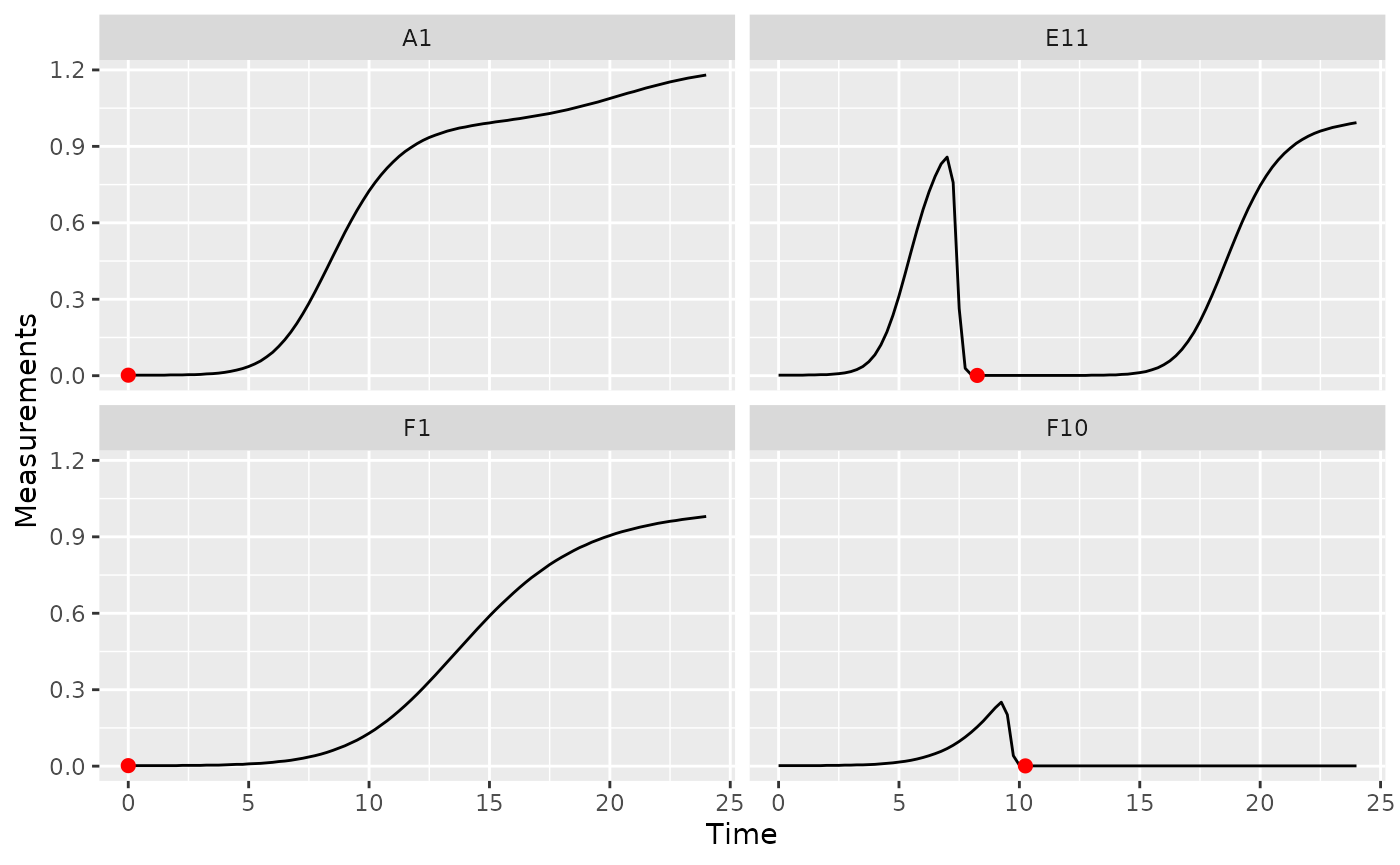In some cases (e.g. growing with phages), bacteria may later drop to a lower density than they started in the growth curve. In this case, we want the first local minima of the Measurements data, rather than the global minima:

ex_dat_mrg_sum <-
summarize(group_by(ex_dat_mrg, Bacteria_strain, Phage, Well),
min_dens = first_minima(y = Measurements, x = Time, return = "y"),
min_time = first_minima(y = Measurements, x = Time, return = "x"))
#> summarise() has grouped output by 'Bacteria_strain', 'Phage'. You can
#> override using the .groups argument.
#> # A tibble: 6 × 5
#> # Groups:   Bacteria_strain, Phage 
#>   Bacteria_strain Phage       Well  min_dens min_time
#>   <chr>           <chr>       <fct>    <dbl>    <dbl>
#> 1 Strain 1        No Phage    A1       0.002        0
#> 2 Strain 1        Phage Added A7       0.002        0
#> 3 Strain 10       No Phage    B4       0.002        0
#> 4 Strain 10       Phage Added B10      0.002        0
#> 5 Strain 11       No Phage    B5       0.002        0
#> 6 Strain 11       Phage Added B11      0.002        0

Note that you can tune the sensitivity of first_minima to different heights and widths of peaks and valleys using the window_width, window_width_n, and window_height arguments. You should check that first_minima is working with your data by plotting it, although the default sensitivity works much of the time.

## Lag time

Bacteria often have a period of time before they reach their maximum growth rate. If you would like to quantify this lag time, you can use the lag_time function. lag_time needs the x and y values, as well as the (per-capita) derivative. It will find the maximum derivative, then project the tangent line with that slope back until it crosses the starting density.

Below, I calculate lag time. So that you can see a visualization of what this tangent-line calculation does, I also calculate the max_percap, max_percap_time, max_percap_dens, and min_dens, but you don’t have to do that.

ex_dat_mrg_sum <-
summarize(group_by(ex_dat_mrg, Bacteria_strain, Phage, Well),
lag_time = lag_time(y = Measurements, x = Time,
deriv = deriv_percap5),
max_percap = max_gc(deriv_percap5),
max_percap_time = Time[which_max_gc(deriv_percap5)],
max_percap_dens = Measurements[which_max_gc(deriv_percap5)],
min_dens = min_gc(Measurements))
#> summarise() has grouped output by 'Bacteria_strain', 'Phage'. You can
#> override using the .groups argument.
#> # A tibble: 6 × 8
#> # Groups:   Bacteria_strain, Phage 
#>   Bacteria_strain Phage       Well  lag_time max_percap max_percap_time
#>   <chr>           <chr>       <fct>    <dbl>      <dbl>           <dbl>
#> 1 Strain 1        No Phage    A1        2.18       1.03            4.25
#> 2 Strain 1        Phage Added A7        1.51       1.03            4.25
#> 3 Strain 10       No Phage    B4        1.78       1.59            3.5
#> 4 Strain 10       Phage Added B10       1.34       1.59            3.5
#> 5 Strain 11       No Phage    B5        1.67       1.65            3.5
#> 6 Strain 11       Phage Added B11       1.24       1.65            3.5
#> # ℹ 2 more variables: max_percap_dens <dbl>, min_dens <dbl>

ggplot(data = dplyr::filter(ex_dat_mrg, Well %in% sample_wells),
aes(x = Time, y = log(Measurements))) +
geom_point() +
facet_wrap(~Well) +
geom_abline(data = dplyr::filter(ex_dat_mrg_sum, Well %in% sample_wells),
color = "red",
aes(slope = max_percap,
intercept = log(max_percap_dens) - max_percap*max_percap_time)) +
geom_vline(data = dplyr::filter(ex_dat_mrg_sum, Well %in% sample_wells),
aes(xintercept = lag_time), lty = 2) +
geom_hline(data = dplyr::filter(ex_dat_mrg_sum, Well %in% sample_wells),
aes(yintercept = log(min_dens)))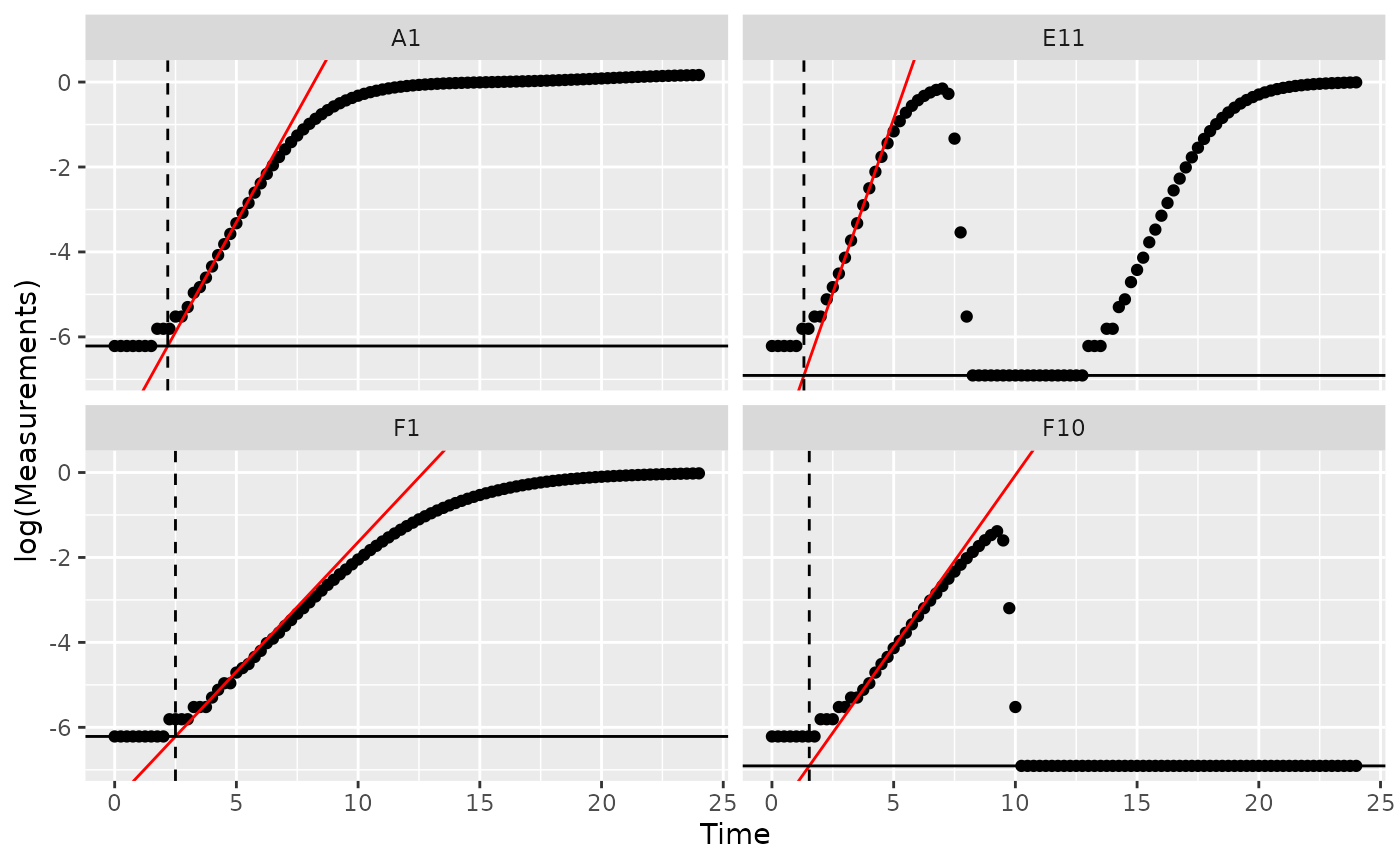Notice how in some of the wells the minimum density value isn’t the initial density? We can fix that by overriding the default minimum density calculation with first_minima via the y0 argument of lag_time.

ex_dat_mrg_sum <-
summarize(group_by(ex_dat_mrg, Bacteria_strain, Phage, Well),
min_dens = first_minima(Measurements, return = "y"),
lag_time = lag_time(y = Measurements, x = Time,
deriv = deriv_percap5, y0 = min_dens),
max_percap = max_gc(deriv_percap5),
max_percap_time = Time[which_max_gc(deriv_percap5)],
max_percap_dens = Measurements[which_max_gc(deriv_percap5)])
#> summarise() has grouped output by 'Bacteria_strain', 'Phage'. You can
#> override using the .groups argument.
#> # A tibble: 6 × 8
#> # Groups:   Bacteria_strain, Phage 
#>   Bacteria_strain Phage       Well  min_dens lag_time max_percap max_percap_time
#>   <chr>           <chr>       <fct>    <dbl>    <dbl>      <dbl>           <dbl>
#> 1 Strain 1        No Phage    A1       0.002     2.18       1.03            4.25
#> 2 Strain 1        Phage Added A7       0.002     2.18       1.03            4.25
#> 3 Strain 10       No Phage    B4       0.002     1.78       1.59            3.5
#> 4 Strain 10       Phage Added B10      0.002     1.78       1.59            3.5
#> 5 Strain 11       No Phage    B5       0.002     1.67       1.65            3.5
#> 6 Strain 11       Phage Added B11      0.002     1.67       1.65            3.5
#> # ℹ 1 more variable: max_percap_dens <dbl>

ggplot(data = dplyr::filter(ex_dat_mrg, Well %in% sample_wells),
aes(x = Time, y = log(Measurements))) +
geom_point() +
facet_wrap(~Well) +
geom_abline(data = dplyr::filter(ex_dat_mrg_sum, Well %in% sample_wells),
color = "red",
aes(slope = max_percap,
intercept = log(max_percap_dens) - max_percap*max_percap_time)) +
geom_vline(data = dplyr::filter(ex_dat_mrg_sum, Well %in% sample_wells),
aes(xintercept = lag_time), lty = 2) +
geom_hline(data = dplyr::filter(ex_dat_mrg_sum, Well %in% sample_wells),
aes(yintercept = log(min_dens)))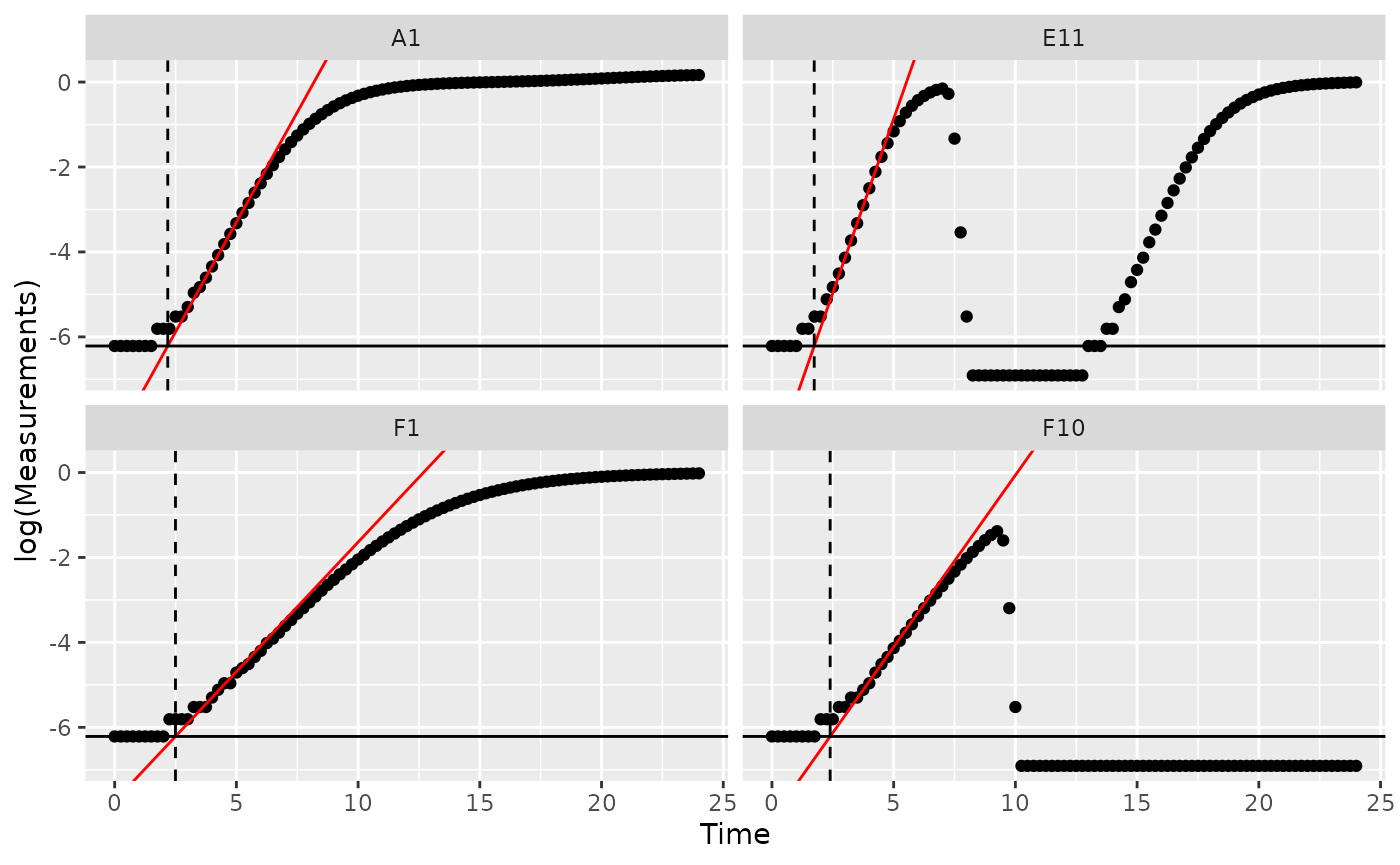## Time to reach threshold density

If you want to quantify how long it takes bacteria to reach some threshold density, you can use the first_above function. In this example, we’ll use a Measurements value of 0.1 as our threshold.

ex_dat_mrg_sum <-
summarize(group_by(ex_dat_mrg, Bacteria_strain, Phage, Well),
above_01 = first_above(y = Measurements, x = Time,
threshold = 0.1, return = "x"))
#> summarise() has grouped output by 'Bacteria_strain', 'Phage'. You can
#> override using the .groups argument.
#> # A tibble: 6 × 4
#> # Groups:   Bacteria_strain, Phage 
#>   Bacteria_strain Phage       Well  above_01
#>   <chr>           <chr>       <fct>    <dbl>
#> 1 Strain 1        No Phage    A1        6.09
#> 2 Strain 1        Phage Added A7        6.09
#> 3 Strain 10       No Phage    B4        4.25
#> 4 Strain 10       Phage Added B10       4.25
#> 5 Strain 11       No Phage    B5        4.04
#> 6 Strain 11       Phage Added B11       4.04

ggplot(data = dplyr::filter(ex_dat_mrg, Well %in% sample_wells),
aes(x = Time, y = Measurements)) +
geom_line() +
facet_wrap(~Well) +
geom_vline(data = dplyr::filter(ex_dat_mrg_sum, Well %in% sample_wells),
aes(xintercept = above_01), lty = 2, color = "red")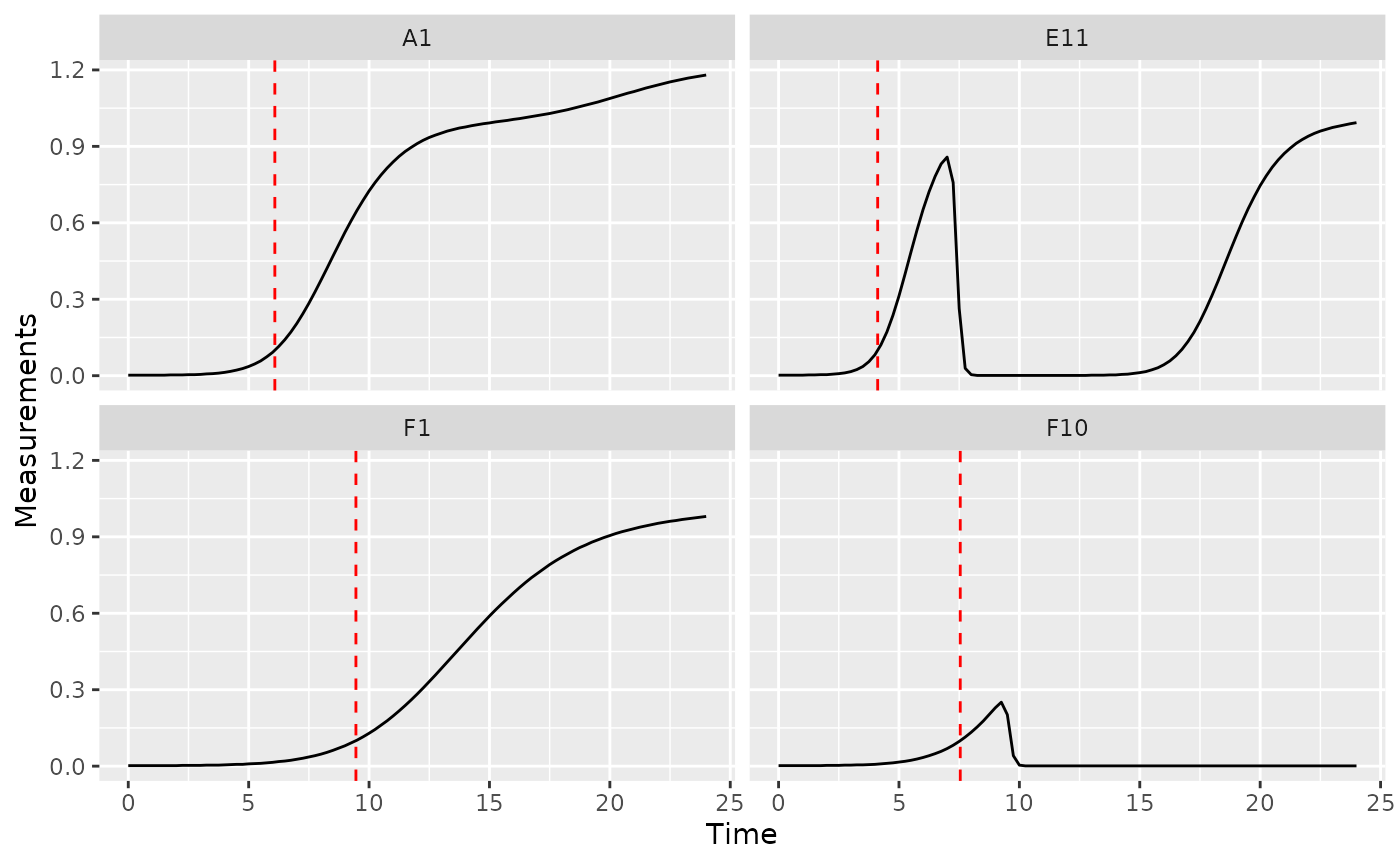## Time to reach threshold growth rate

If you want to quantify how long it takes bacteria to reach some threshold per-capita growth rate, you can use the first_above function. In this example, we’ll use a per-capita derivative of 1 as our threshold.

ex_dat_mrg_sum <-
summarize(group_by(ex_dat_mrg, Bacteria_strain, Phage, Well),
percap_above_1 = first_above(y = deriv_percap5, x = Time,
threshold = 1, return = "x"))
#> summarise() has grouped output by 'Bacteria_strain', 'Phage'. You can
#> override using the .groups argument.
#> # A tibble: 6 × 4
#> # Groups:   Bacteria_strain, Phage 
#>   Bacteria_strain Phage       Well  percap_above_1
#>   <chr>           <chr>       <fct>          <dbl>
#> 1 Strain 1        No Phage    A1              3.95
#> 2 Strain 1        Phage Added A7              3.95
#> 3 Strain 10       No Phage    B4              2.28
#> 4 Strain 10       Phage Added B10             2.28
#> 5 Strain 11       No Phage    B5              2.11
#> 6 Strain 11       Phage Added B11             2.11

ggplot(data = dplyr::filter(ex_dat_mrg, Well %in% sample_wells),
aes(x = Time, y = deriv_percap5)) +
geom_line() +
facet_wrap(~Well) +
geom_vline(data = dplyr::filter(ex_dat_mrg_sum, Well %in% sample_wells),
aes(xintercept = percap_above_1), lty = 2, color = "red") +
coord_cartesian(ylim = c(-1, NA))
#> Warning: Removed 4 rows containing missing values (geom_line()).
#> Warning: Removed 2 rows containing missing values (geom_vline()).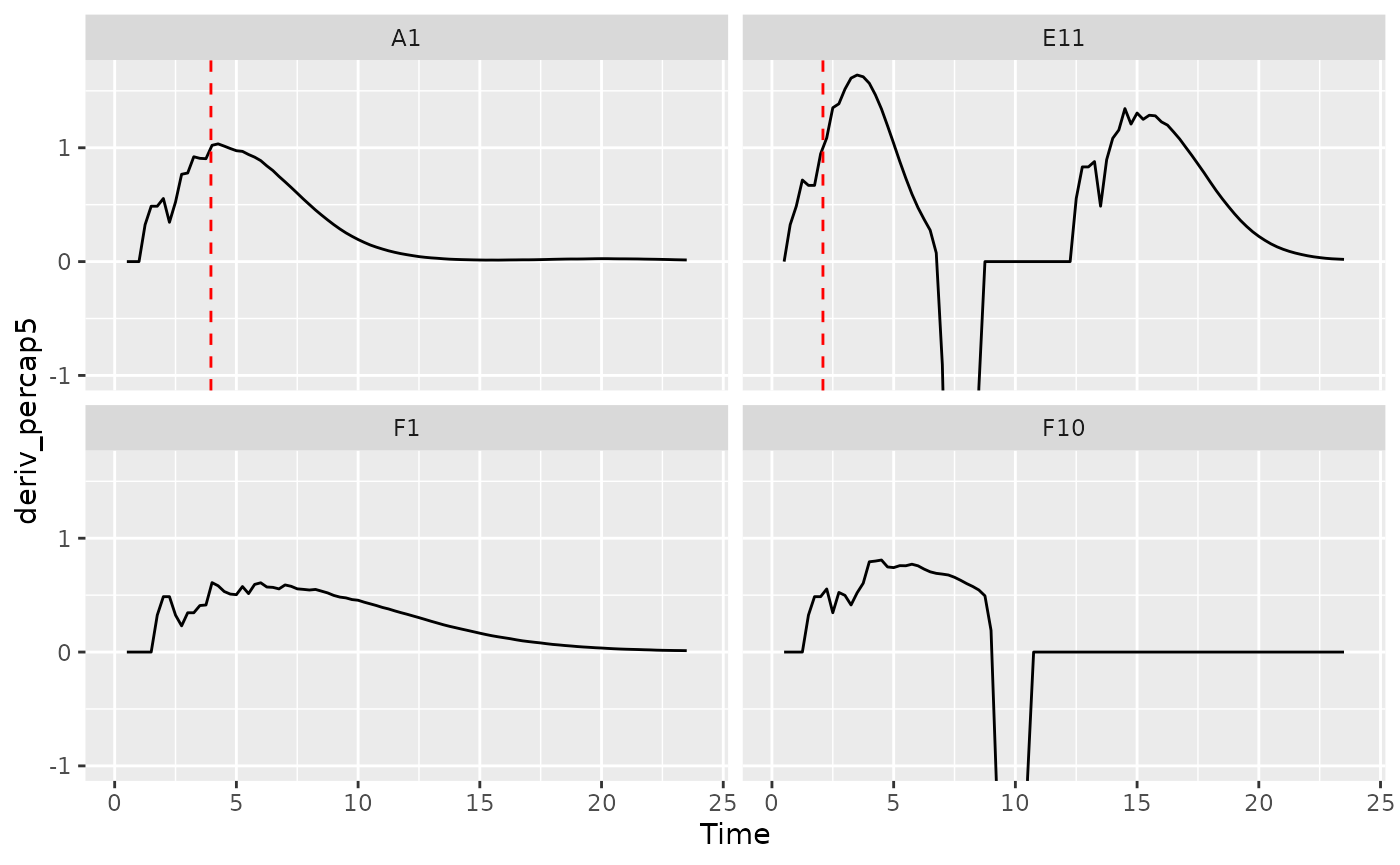## Maximum growth rate and minimum doubling time

If you want to calculate the bacterial maximum growth rate (i.e. the minimum doubling time), it will often be sufficient to use max_gc on the per-capita derivatives we calculated in vignette("process"). (max_gc works just like R’s built-in max, but with better default settings for growth curve analyses with summarize).

We can also save the time when this maximum occurs using the which_max_gc function. which_max_gc returns the index of the maximum value, so then we can get the Time value at that index and save it to a column titled max_percap_time. (which_max_gc and extr_val work just like R’s built-in which.max and [, but with better default settings for growth curve analyses with summarize)

If you would like the equivalent minimum doubling time, you can simply calculate the maximum growth rate as above and then convert that into the equivalent minimum doubling time using the doubling_time function.

ex_dat_mrg_sum <-
summarize(group_by(ex_dat_mrg, Bacteria_strain, Phage, Well),
max_percap = max_gc(deriv_percap5, na.rm = TRUE),
max_percap_time = extr_val(Time, which_max_gc(deriv_percap5)),
doub_time = doubling_time(y = max_percap))
#> summarise() has grouped output by 'Bacteria_strain', 'Phage'. You can
#> override using the .groups argument.
#> # A tibble: 6 × 6
#> # Groups:   Bacteria_strain, Phage 
#>   Bacteria_strain Phage       Well  max_percap max_percap_time doub_time
#>   <chr>           <chr>       <fct>      <dbl>           <dbl>     <dbl>
#> 1 Strain 1        No Phage    A1          1.03            4.25     0.670
#> 2 Strain 1        Phage Added A7          1.03            4.25     0.670
#> 3 Strain 10       No Phage    B4          1.59            3.5      0.436
#> 4 Strain 10       Phage Added B10         1.59            3.5      0.436
#> 5 Strain 11       No Phage    B5          1.65            3.5      0.421
#> 6 Strain 11       Phage Added B11         1.65            3.5      0.421

ggplot(data = dplyr::filter(ex_dat_mrg, Well %in% sample_wells),
aes(x = Time, y = deriv_percap5)) +
geom_line() +
facet_wrap(~Well) +
geom_point(data = dplyr::filter(ex_dat_mrg_sum, Well %in% sample_wells),
aes(x = max_percap_time, y = max_percap),
size = 2, color = "red") +
coord_cartesian(ylim = c(-1, NA))
#> Warning: Removed 4 rows containing missing values (geom_line()).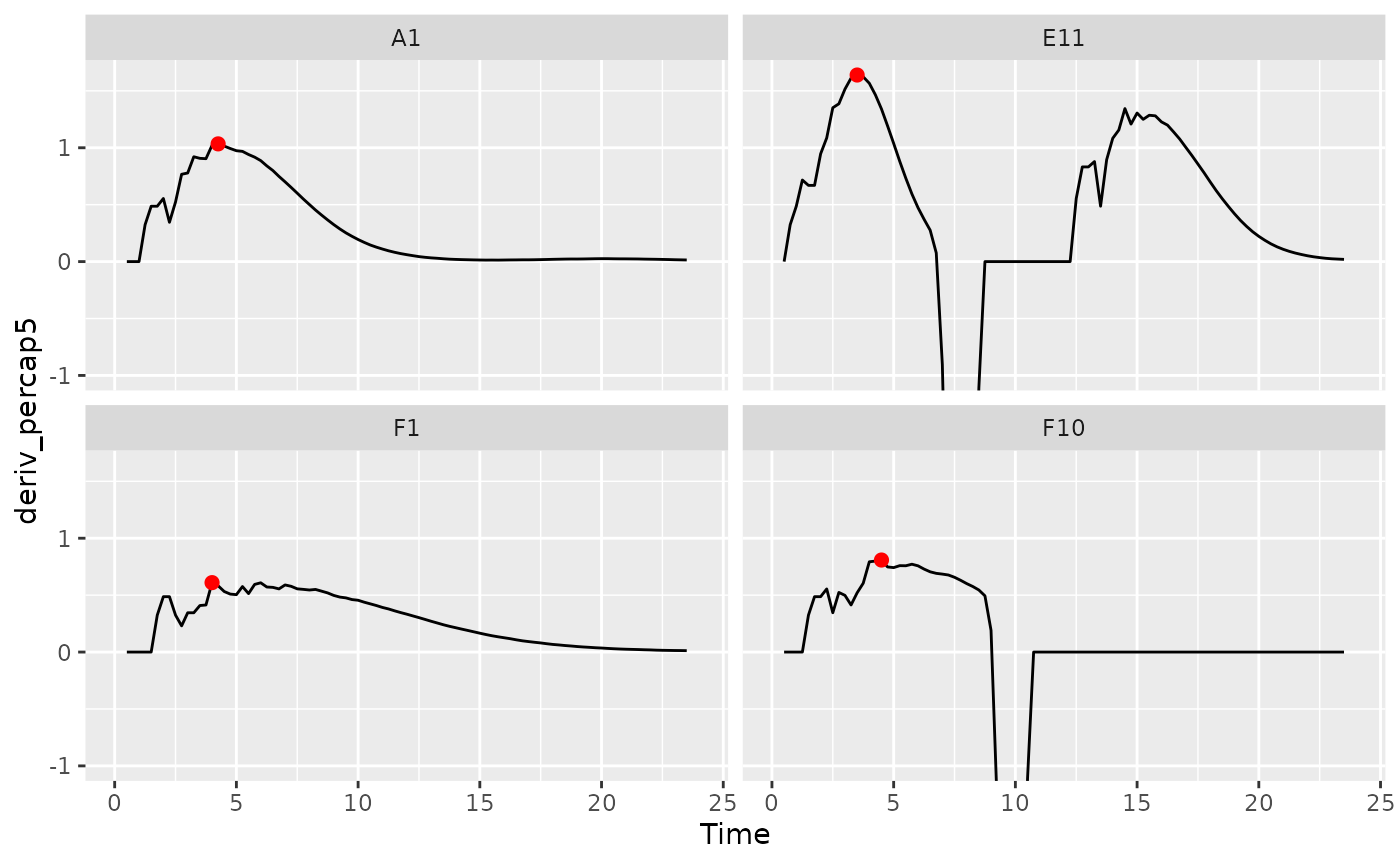## Mid-point time or inflection point

If you want to find the mid-point or inflection point of bacterial growth, there are two different approaches:

1. Mid-point: find the point when the density first reaches half the maximum density.
2. Inflection point: find the point when the derivative is at a maximum.

In growth curve analysis approaches using fitting of a symmetric function (e.g. when other R packages fit a logistic function to data), these two points will be equivalent. However, since gcplyr does model-free analyses, we do not assume symmetry, and so the points may be very similar or very different.

For the mid-point, we use the first_above function, with the threshold equal to the maximum bacterial density divided by 2. For the inflection point, we find the time when the deriv was at a maximum using which_max_gc (which_max_gc and extr_val work just like R’s built-in which.max and [, but with better default settings for growth curve analyses with summarize).

ex_dat_mrg_sum <-
summarize(group_by(ex_dat_mrg, Bacteria_strain, Phage, Well),
mid_point = first_above(y = Measurements, x = Time, return = "x",
threshold = max_gc(Measurements)/2),
infl_point = extr_val(Time, which_max_gc(deriv)))
#> summarise() has grouped output by 'Bacteria_strain', 'Phage'. You can
#> override using the .groups argument.
#> # A tibble: 6 × 5
#> # Groups:   Bacteria_strain, Phage 
#>   Bacteria_strain Phage       Well  mid_point infl_point
#>   <chr>           <chr>       <fct>     <dbl>      <dbl>
#> 1 Strain 1        No Phage    A1         9.15       8.25
#> 2 Strain 1        Phage Added A7         7.29       8.25
#> 3 Strain 10       No Phage    B4         6.06       5.5
#> 4 Strain 10       Phage Added B10        5.67       5.5
#> 5 Strain 11       No Phage    B5         5.71       5
#> 6 Strain 11       Phage Added B11       16.7        5

ggplot(data = dplyr::filter(ex_dat_mrg, Well %in% sample_wells),
aes(x = Time, y = Measurements)) +
geom_line() +
facet_wrap(~Well) +
geom_vline(data = dplyr::filter(ex_dat_mrg_sum, Well %in% sample_wells),
aes(xintercept = mid_point), lty = 2, color = "red") +
geom_vline(data = dplyr::filter(ex_dat_mrg_sum, Well %in% sample_wells),
aes(xintercept = infl_point), lty = 2, color = "blue")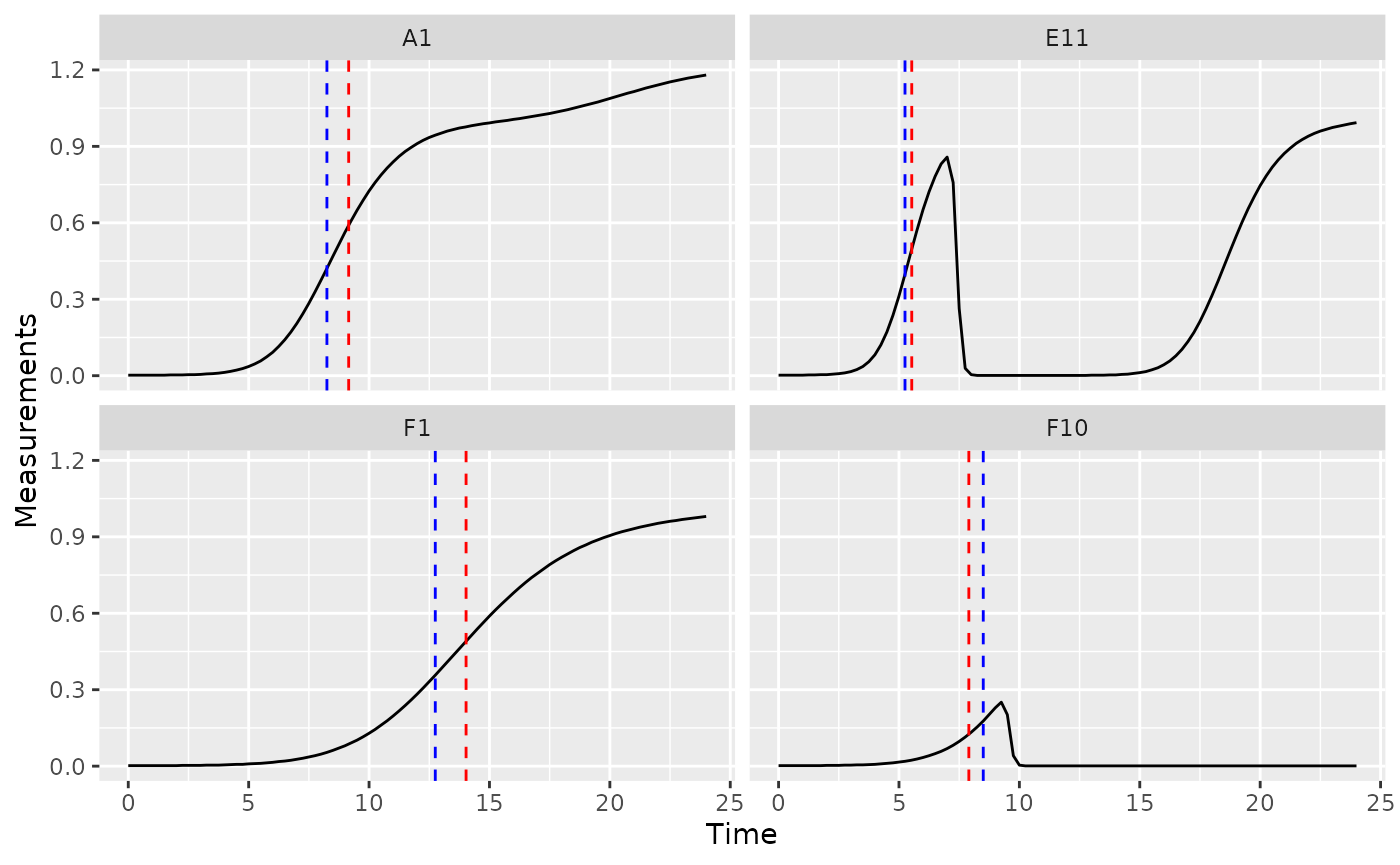## Maximum density

The maximum bacterial density can be a measure of bacterial carrying capacity in a given media or as a measure of bacterial growth yield/efficiency. If you want to quantify the maximum bacterial density, we can use max_gc to get the global maxima of Measurements (max_gc, which_max_gc, and extr_val work just like R’s built-in max, which.max, and [, but with better default settings for growth curve analyses with summarize).

See Peak bacterial density for identifying local maxima of Measurements (e.g. if you wanted the first peak in Well E11 shown below).

ex_dat_mrg_sum <-
summarize(group_by(ex_dat_mrg, Bacteria_strain, Phage, Well),
max_dens = max_gc(Measurements, na.rm = TRUE),
max_time = extr_val(Time, which_max_gc(Measurements)))
#> summarise() has grouped output by 'Bacteria_strain', 'Phage'. You can
#> override using the .groups argument.
#> # A tibble: 6 × 5
#> # Groups:   Bacteria_strain, Phage 
#>   Bacteria_strain Phage       Well  max_dens max_time
#>   <chr>           <chr>       <fct>    <dbl>    <dbl>
#> 1 Strain 1        No Phage    A1       1.18     24
#> 2 Strain 1        Phage Added A7       0.499     8.75
#> 3 Strain 10       No Phage    B4       1.21     23.8
#> 4 Strain 10       Phage Added B10      0.962     8.5
#> 5 Strain 11       No Phage    B5       1.21     19.5
#> 6 Strain 11       Phage Added B11      1.03     24

ggplot(data = dplyr::filter(ex_dat_mrg, Well %in% sample_wells),
aes(x = Time, y = Measurements)) +
geom_line() +
facet_wrap(~Well) +
geom_point(data = dplyr::filter(ex_dat_mrg_sum, Well %in% sample_wells),
aes(x = max_time, y = max_dens),
size = 2, color = "red")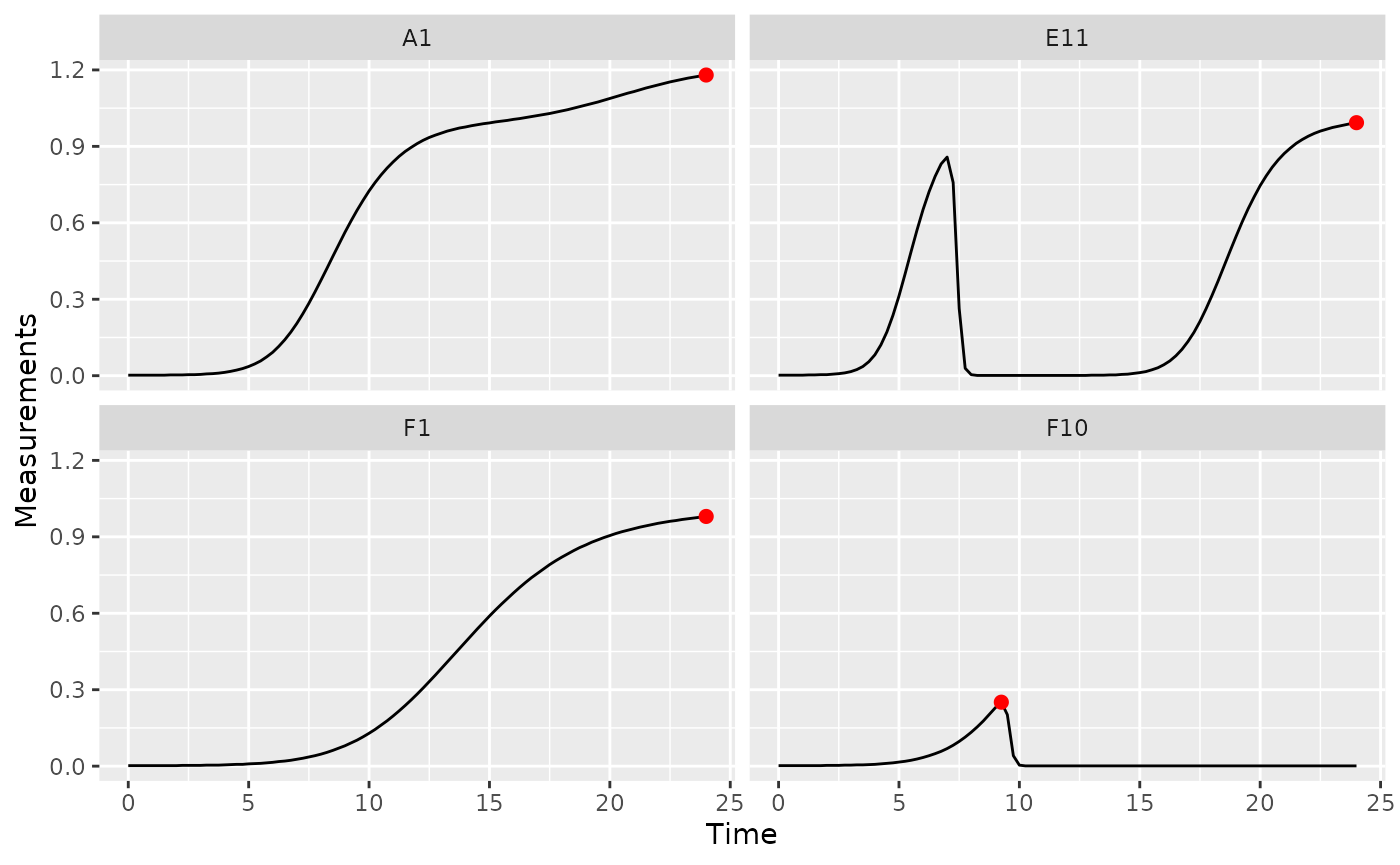## Area under the curve

The area under the curve is a common metric of total bacterial growth, for instance in the presence of antagonists like antibiotics or phages. If you want to calculate the area under the curve, you can use the gcplyr function auc. Simply specify Time as the x and Measurements as the y data whose area-under-the-curve you want to calculate.

ex_dat_mrg_sum <-
summarize(group_by(ex_dat_mrg, Bacteria_strain, Phage, Well),
auc = auc(x = Time, y = Measurements))
#> summarise() has grouped output by 'Bacteria_strain', 'Phage'. You can
#> override using the .groups argument.
#> # A tibble: 6 × 4
#> # Groups:   Bacteria_strain, Phage 
#>   Bacteria_strain Phage       Well    auc
#>   <chr>           <chr>       <fct> <dbl>
#> 1 Strain 1        No Phage    A1    15.9
#> 2 Strain 1        Phage Added A7     1.07
#> 3 Strain 10       No Phage    B4    20.4
#> 4 Strain 10       Phage Added B10    6.15
#> 5 Strain 11       No Phage    B5    20.9
#> 6 Strain 11       Phage Added B11    7.77

## Diauxic shifts

Bacteria frequently exhibit a second, slower, burst of growth after their first period of rapid growth. This is common in growth curves and is called diauxic growth.

If we plot the data from some of our example data wells with no phage added, we’ll see this pattern repeatedly:

nophage_wells <- c("A1", "A4", "E2", "F1")
ggplot(data = dplyr::filter(ex_dat_mrg, Well %in% nophage_wells),
aes(x = Time, y = Measurements)) +
geom_line() +
facet_wrap(~Well, scales = "free")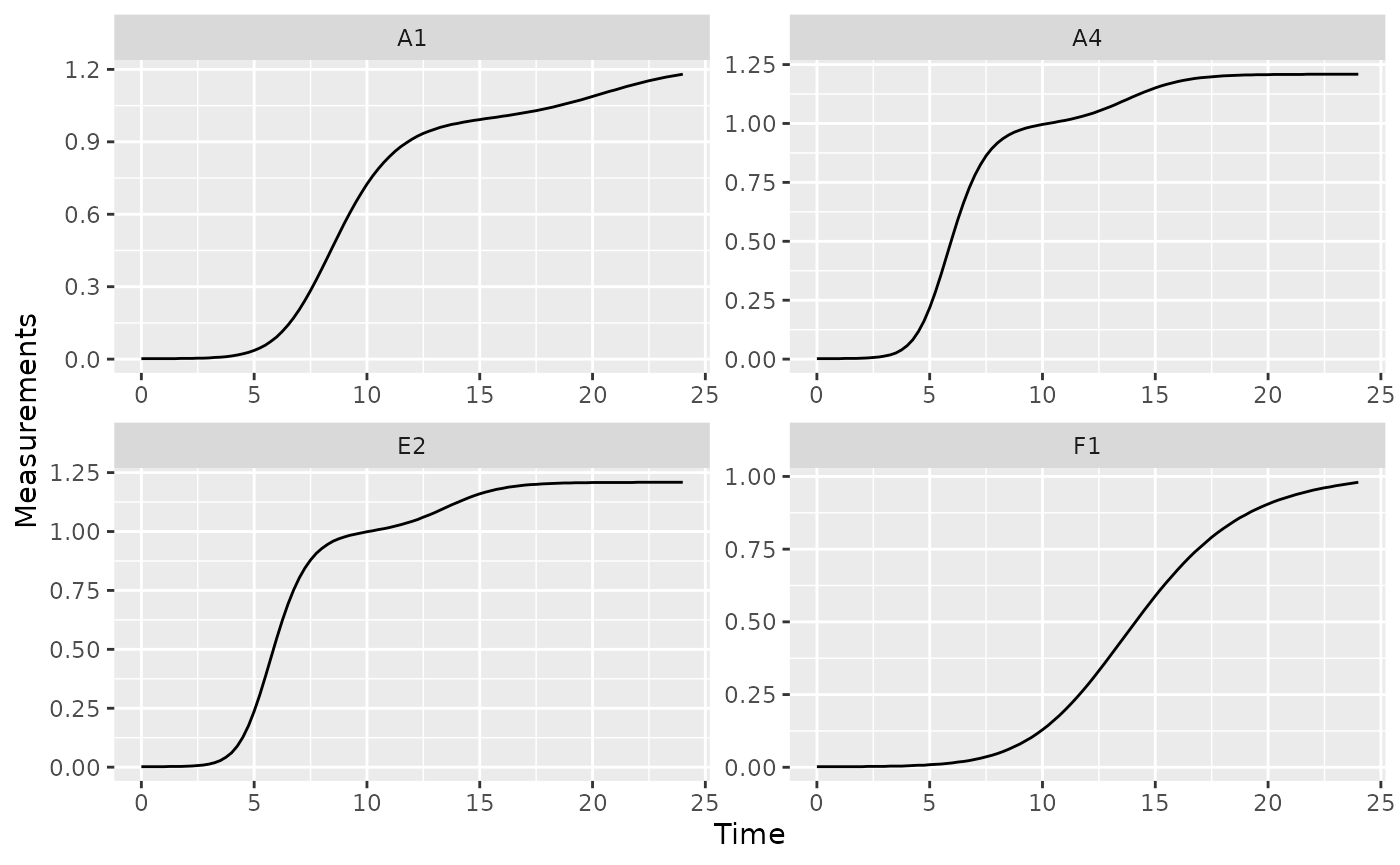We can identify the time when bacteria switch from their first period of rapid growth to their second period by finding a minima in the derivative values. Specifically, we want to identify the second minima (the first minima will occur at the beginning of the growth curve, when bacteria are just starting to grow). Let’s look at some of the derivative values to see this.

ggplot(data = dplyr::filter(ex_dat_mrg, Well %in% nophage_wells),
aes(x = Time, y = deriv)) +
geom_line() +
facet_wrap(~Well, scales = "free")
#> Warning: Removed 1 row containing missing values (geom_line()).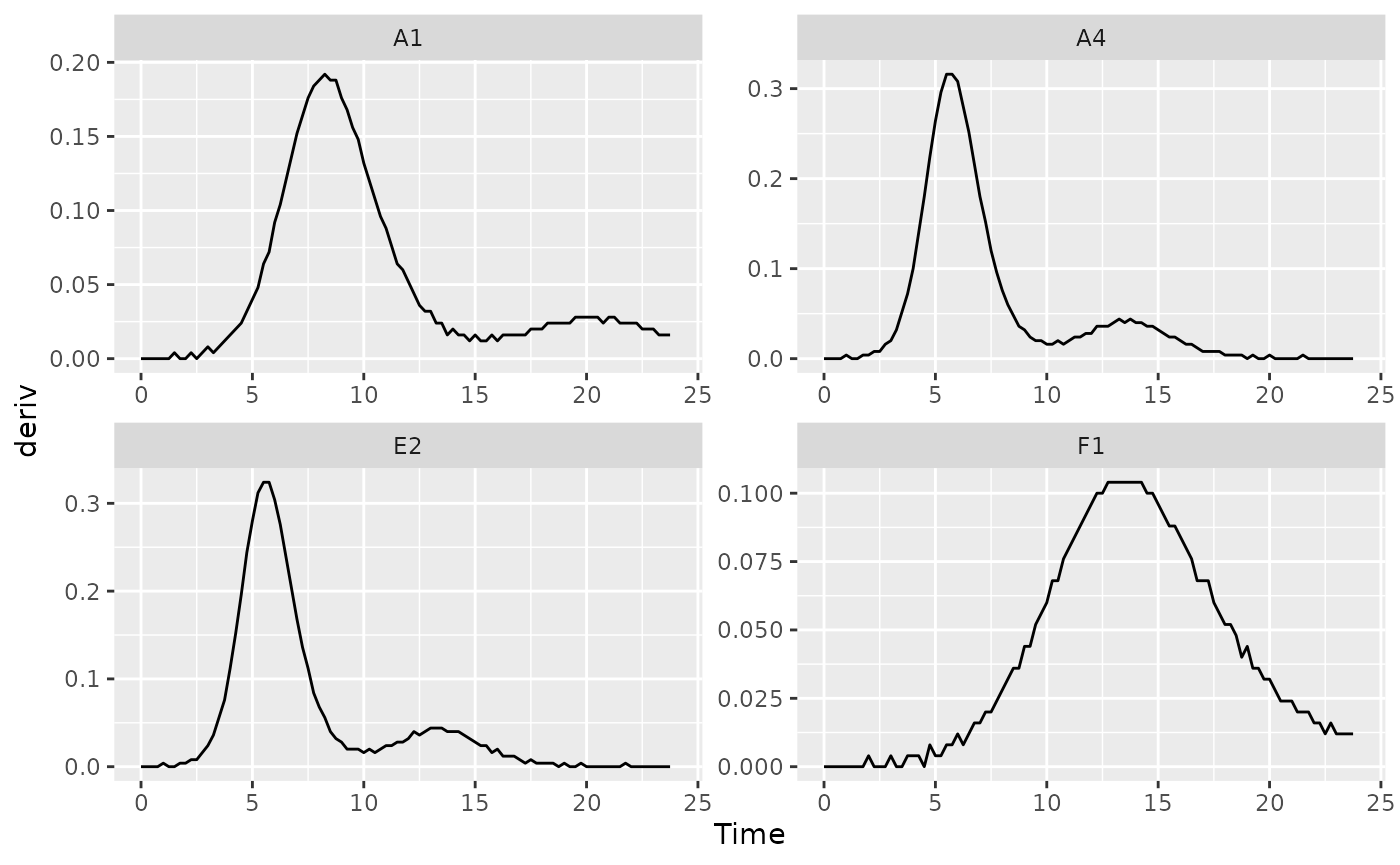We can use the gcplyr function find_local_extrema to find that minima. Specify deriv as the y data and Time as the x data, and that we want find_local_extrema to return the x values associated with local minima. It will return a vector of those x values, and we’re going to save just the second one.

At the same time, we’re also going to save the density where the diauxic shift occurs. First, we’ll use find_local_extrema again, but this time to save the index where the diauxic shift occurs to a column titled diauxie_idx. Then, we can get the Measurements value at that index. (Note that it wouldn’t work to just specify return = "y", because the y values in this case are the deriv values). (extr_val works just like R’s built-in [, but with better default settings for growth curve analyses with summarize).

ex_dat_mrg_sum <-
summarize(group_by(ex_dat_mrg, Bacteria_strain, Phage, Well),
diauxie_time = find_local_extrema(x = Time, y = deriv, return = "x",
return_maxima = FALSE, return_minima = TRUE,
window_width_n = 39),
diauxie_idx = find_local_extrema(x = Time, y = deriv, return = "index",
return_maxima = FALSE, return_minima = TRUE,
window_width_n = 39),
diauxie_dens = extr_val(Measurements, diauxie_idx))
#> summarise() has grouped output by 'Bacteria_strain', 'Phage'. You can
#> override using the .groups argument.
#> # A tibble: 6 × 6
#> # Groups:   Bacteria_strain, Phage 
#>   Bacteria_strain Phage       Well  diauxie_time diauxie_idx diauxie_dens
#>   <chr>           <chr>       <fct>        <dbl>       <int>        <dbl>
#> 1 Strain 1        No Phage    A1           16             65        1.01
#> 2 Strain 1        Phage Added A7            9             37        0.379
#> 3 Strain 10       No Phage    B4            9.75          40        0.999
#> 4 Strain 10       Phage Added B10           9.25          38        0.682
#> 5 Strain 11       No Phage    B5            9.5           39        1.01
#> 6 Strain 11       Phage Added B11           5.5           23        0.346

# Plot data with a point at the moment of diauxic shift
ggplot(data = dplyr::filter(ex_dat_mrg, Well %in% nophage_wells),
aes(x = Time, y = Measurements)) +
geom_line() +
facet_wrap(~Well, scales = "free") +
geom_point(data = dplyr::filter(ex_dat_mrg_sum, Well %in% nophage_wells),
aes(x = diauxie_time, y = diauxie_dens),
size = 2, color = "red")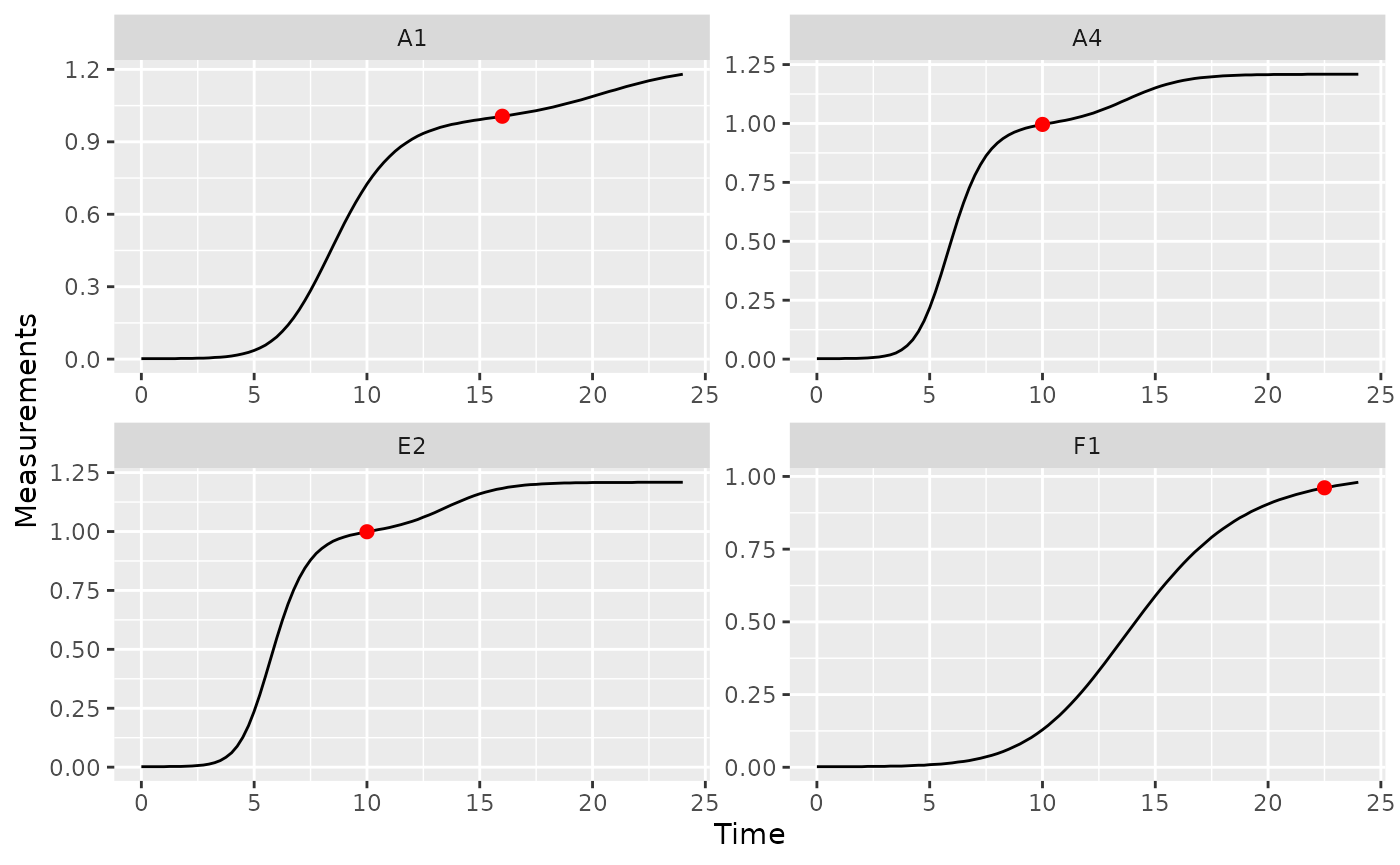If needed, you can tune the sensitivity of find_local_extrema to different heights and widths of peaks and valleys using the window_width, window_width_n, and window_height arguments.

## Growth rate during diauxie

In the previous section we identified when bacteria shifted into their second period of rapid growth (‘diauxic growth’). If you want to find out what the peak per-capita growth rate was during that second burst, we’ll have to use max on the subset of data after the diauxic shift identified by find_local_extrema

Just as we did in the previous section, we’ll use find_local_extrema to save the time when the diauxic shift occurs. Then, we’ll find the maximum of the per-capita derivative after that shift occurs. Finally, we’ll find the time when that post-diauxie maximum growth rate occurs. Note that we’re using max_gc and which_max_gc, which work just like R’s built-in max and which.max, but with better default settings for growth curve analyses with summarize.

ex_dat_mrg_sum <-
summarize(
group_by(ex_dat_mrg, Bacteria_strain, Phage, Well),
diauxie_time =
find_local_extrema(x = Time, y = deriv, return = "x",
return_maxima = FALSE, return_minima = TRUE,
window_width_n = 39),
diauxie_percap = max_gc(deriv_percap5[Time >= diauxie_time]),
diauxie_percap_time =
extr_val(Time[Time >= diauxie_time],
which_max_gc(deriv_percap5[Time >= diauxie_time]))
)
#> summarise() has grouped output by 'Bacteria_strain', 'Phage'. You can
#> override using the .groups argument.
#> # A tibble: 6 × 6
#> # Groups:   Bacteria_strain, Phage 
#>   Bacteria_strain Phage    Well  diauxie_time diauxie_percap diauxie_percap_time
#>   <chr>           <chr>    <fct>        <dbl>          <dbl>               <dbl>
#> 1 Strain 1        No Phage A1           16            0.0257                20
#> 2 Strain 1        Phage A… A7            9            0.832                 19.8
#> 3 Strain 10       No Phage B4            9.75         0.0398                13.2
#> 4 Strain 10       Phage A… B10           9.25         1.24                  17.8
#> 5 Strain 11       No Phage B5            9.5          0.0438                12.2
#> 6 Strain 11       Phage A… B11           5.5          1.43                  12.8

# Plot data with a point at the moment of peak diauxic growth rate
ggplot(data = dplyr::filter(ex_dat_mrg, Well %in% nophage_wells),
aes(x = Time, y = deriv_percap5)) +
geom_line() +
facet_wrap(~Well, scales = "free") +
geom_point(data = dplyr::filter(ex_dat_mrg_sum, Well %in% nophage_wells),
aes(x = diauxie_percap_time, y = diauxie_percap),
size = 2, color = "red")
#> Warning: Removed 4 rows containing missing values (geom_line()).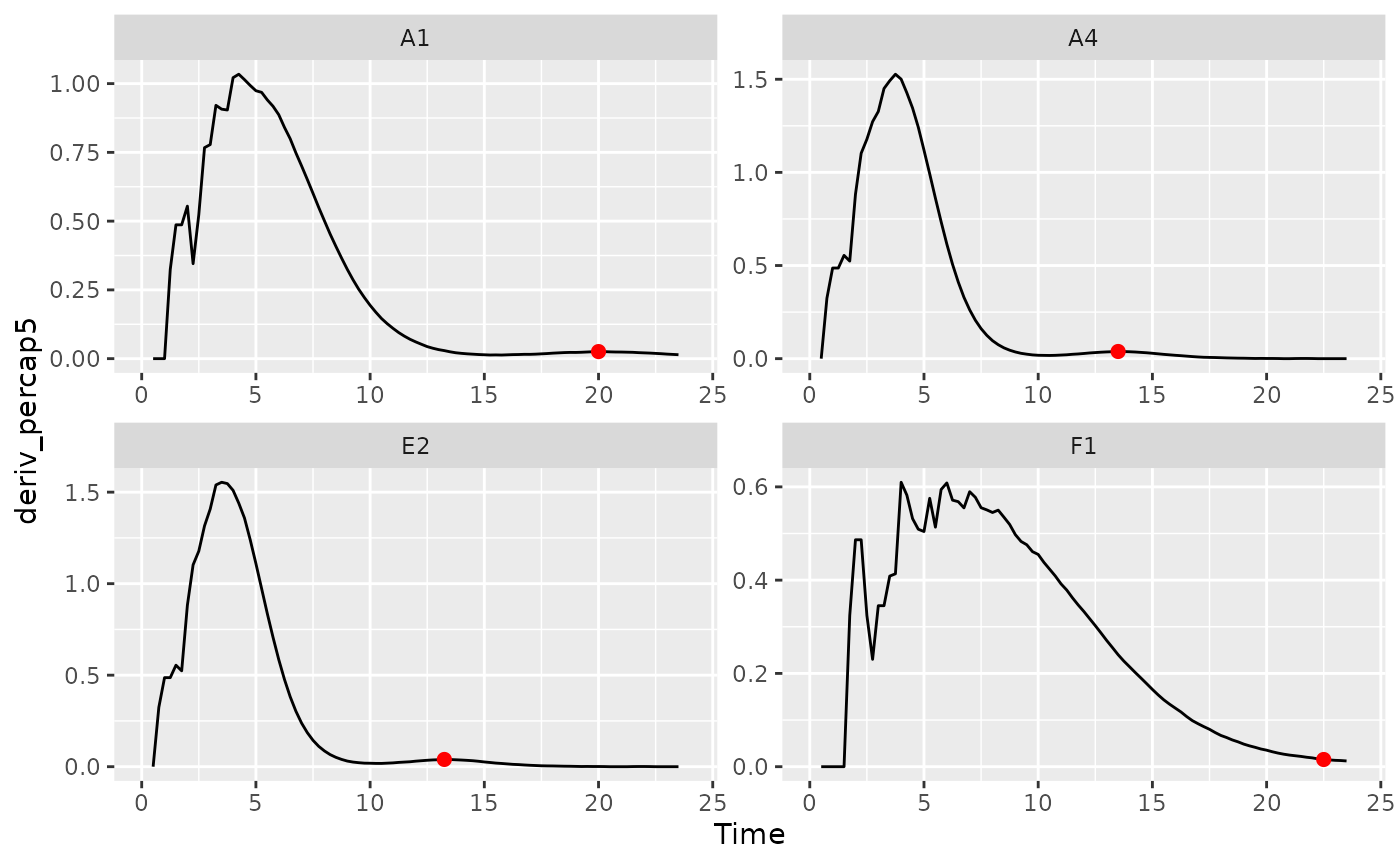## Peak bacterial density

We previously found the global maximum in bacterial density using the simple max_gc and which_max_gc functions. The first local maxima can also be of interest. This is especially true when bacteria are grown with phages, where their first peak density can act as a proxy measure for their susceptibility to the phage. If you’re interested in finding the first local maxima in bacterial density, you can use the gcplyr function first_maxima.

first_maxima simply requires the y data you want to identify the peak in. Specify Measurements as the y data and Time as the x data, and that we want first_peak to return the x and y values associated with the peak. We’ll save those in columns first_maxima_x and first_maxima_y, respectively.

ex_dat_mrg_sum <-
summarize(group_by(ex_dat_mrg, Bacteria_strain, Phage, Well),
first_maxima_x = first_maxima(x = Time, y = Measurements,
return = "x"),
first_maxima_y = first_maxima(x = Time, y = Measurements,
return = "y"))
#> summarise() has grouped output by 'Bacteria_strain', 'Phage'. You can
#> override using the .groups argument.
#> # A tibble: 6 × 5
#> # Groups:   Bacteria_strain, Phage 
#>   Bacteria_strain Phage       Well  first_maxima_x first_maxima_y
#>   <chr>           <chr>       <fct>          <dbl>          <dbl>
#> 1 Strain 1        No Phage    A1             24             1.18
#> 2 Strain 1        Phage Added A7              8.75          0.499
#> 3 Strain 10       No Phage    B4             19.8           1.21
#> 4 Strain 10       Phage Added B10             8.5           0.962
#> 5 Strain 11       No Phage    B5             19.5           1.21
#> 6 Strain 11       Phage Added B11             5.25          0.439

ggplot(data = dplyr::filter(ex_dat_mrg, Well %in% sample_wells),
aes(x = Time, y = Measurements)) +
geom_line() +
facet_wrap(~Well) +
geom_point(data = dplyr::filter(ex_dat_mrg_sum, Well %in% sample_wells),
aes(x = first_maxima_x, y = first_maxima_y),
color = "red", size = 1.5)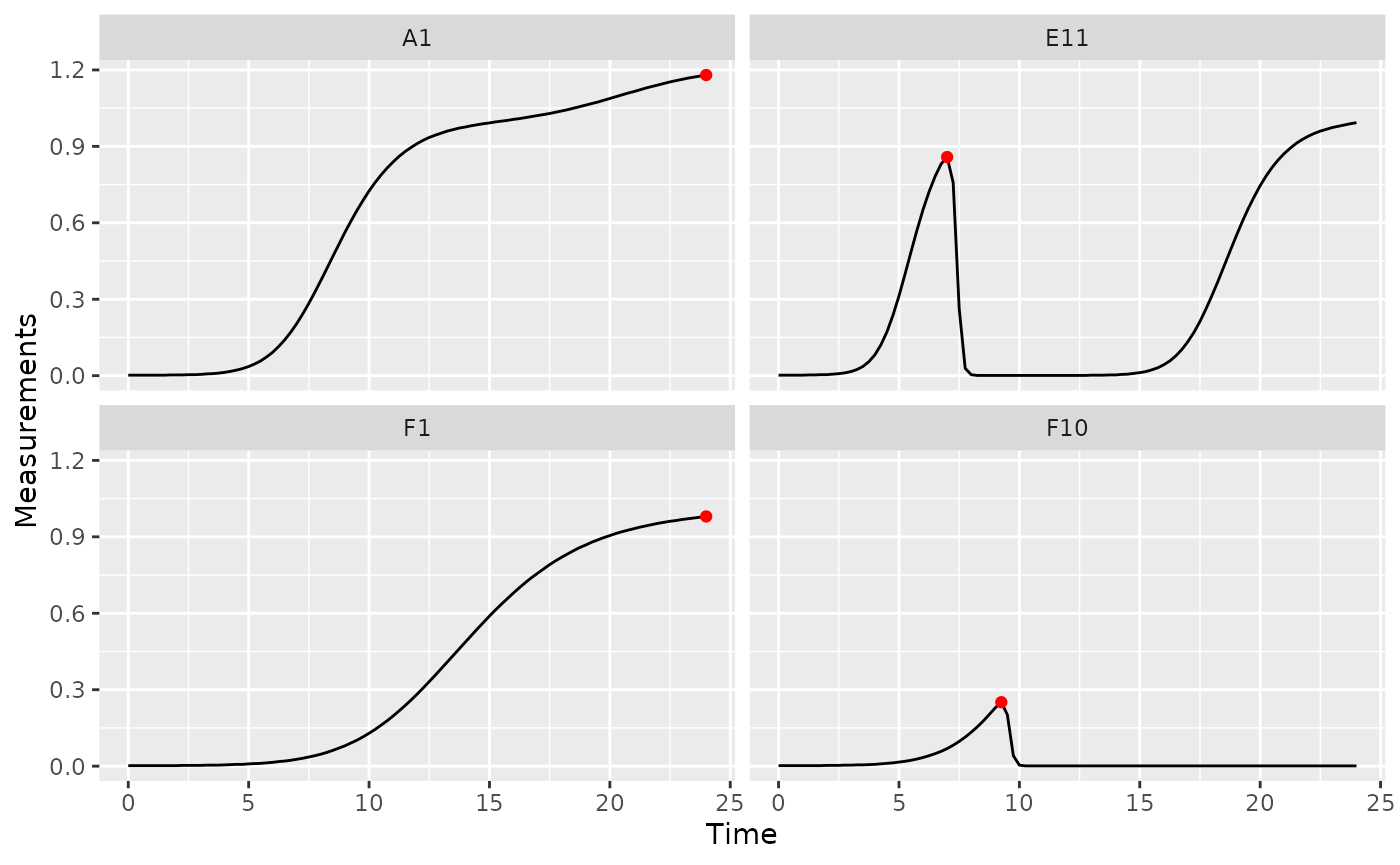Note that you can tune the sensitivity of first_maxima to different heights and widths of peaks and valleys using the window_width, window_width_n, and window_height arguments, although the defaults work much of the time.

## Extinction time

The time when bacterial density falls below some threshold can also be of interest. This is especially true when bacteria are grown with phages, where this ‘extinction time’ can act as a proxy measure for their susceptibility to the phage. If you’re interested in finding the extinction time, you can use the gcplyr function first_below. In this example, we’ll use a Measurements value of 0.15 as our threshold.

ex_dat_mrg_sum <-
summarize(
group_by(ex_dat_mrg, Bacteria_strain, Phage, Well),
extin_time = first_below(x = Time, y = Measurements, threshold = 0.15,
return = "x", return_endpoints = FALSE))
#> summarise() has grouped output by 'Bacteria_strain', 'Phage'. You can
#> override using the .groups argument.
#> # A tibble: 6 × 4
#> # Groups:   Bacteria_strain, Phage 
#>   Bacteria_strain Phage       Well  extin_time
#>   <chr>           <chr>       <fct>      <dbl>
#> 1 Strain 1        No Phage    A1         NA
#> 2 Strain 1        Phage Added A7          9.18
#> 3 Strain 10       No Phage    B4         NA
#> 4 Strain 10       Phage Added B10         9.71
#> 5 Strain 11       No Phage    B5         NA
#> 6 Strain 11       Phage Added B11         5.64

phage_wells <- c("A7", "B10", "F10", "H8")
ggplot(data = dplyr::filter(ex_dat_mrg, Well %in% phage_wells),
aes(x = Time, y = Measurements)) +
geom_line() +
facet_wrap(~Well) +
geom_vline(data = dplyr::filter(ex_dat_mrg_sum, Well %in% phage_wells),
aes(xintercept = extin_time), lty = 2)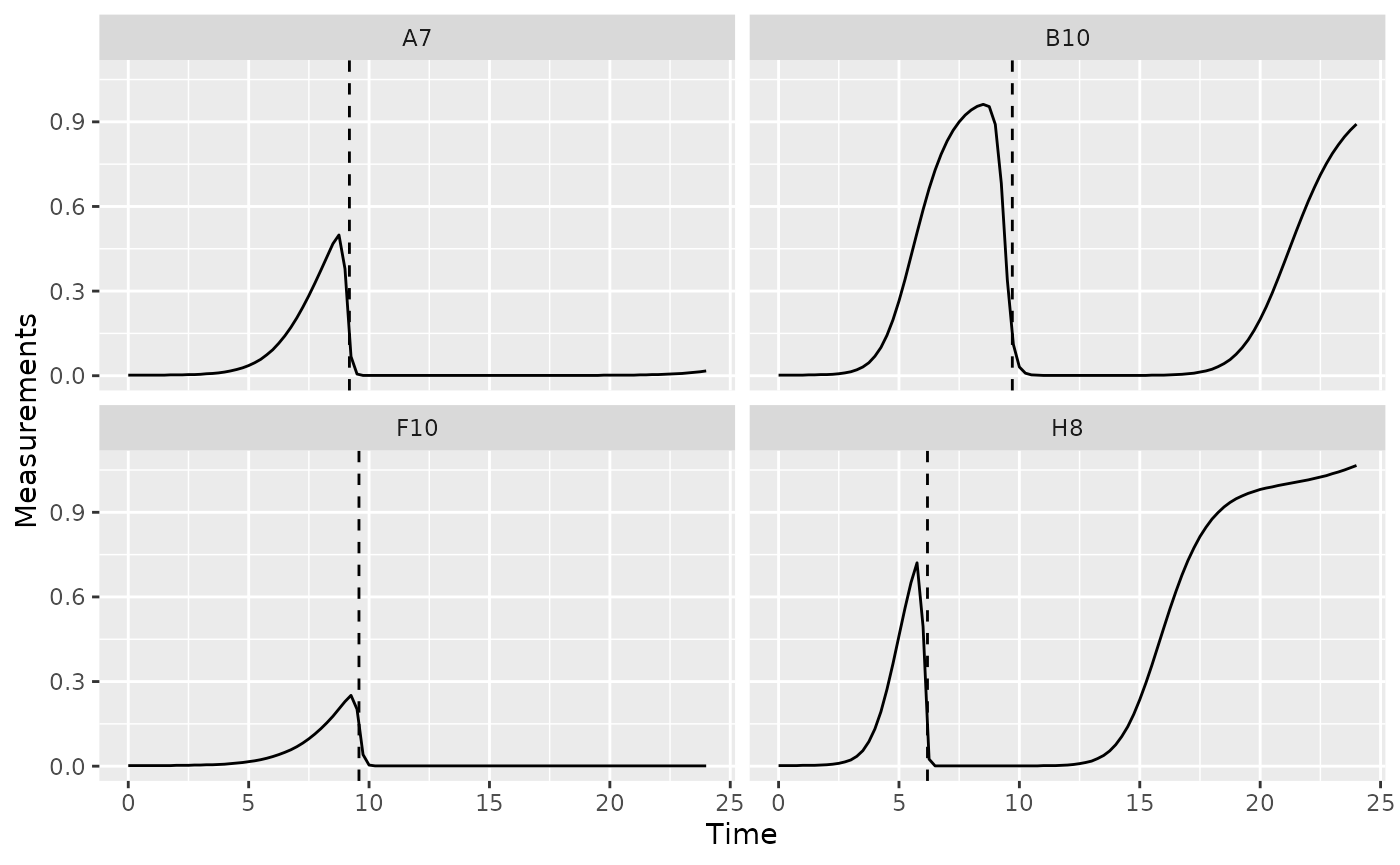## What’s next?

Now that you’ve analyzed your data, you can read about approaches to deal with noise in your growth curve data, or you can read some concluding notes on best practices for running statistics, merging growth curve analyses with other data, and additional resources for analyzing growth curves.

1. Introduction: vignette("gcplyr")
2. Importing and transforming data: vignette("import_transform")
3. Incorporating design information: vignette("incorporate_designs")
4. Pre-processing and plotting your data: vignette("preprocess_plot")
5. Processing your data: vignette("process")
6. Analyzing your data: vignette("analyze")
7. Dealing with noise: vignette("noise")
8. Statistics, merging other data, and other resources: vignette("conclusion")